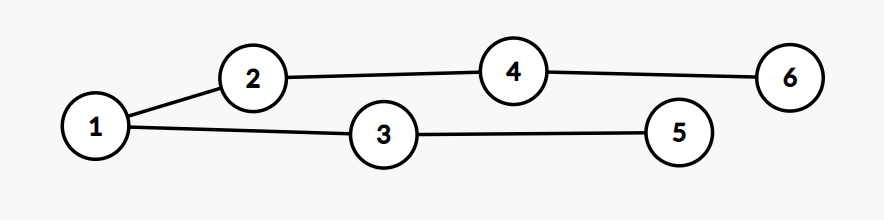## 【树】2020ICPC小米网赛1G-Tree Projection

### 传送门

top：6 4 2 1 3 5

dfs：1 2 3 4 5 6#include<bits/stdc++.h>
using namespace std;
typedef long long LL;
typedef pair<int,int> pii;
mt19937 mrand(random_device{}());
int rnd(int x) { return mrand() % x;}
const int N = 2e5 + 233;
int a[N], b[N], pos[N];
int main()
{
// time_t startt = clock();

// cin.tie(0); cout.tie(0); ios::sync_with_stdio(0);
int n; cin >> n;
for(int i = 1; i <= n; i++) cin >> a[i], pos[a[i]] = i;
for(int i = 1; i <= n; i++) cin >> b[i];

puts("YES");
for(int i = 2, j = b; i <= n; i++)
{
printf("%d %d\n", b[i], j);
if(pos[b[i]] < pos[j]) j = b[i];
}

// cerr << "~ #" << " done! time : " << (double)(clock()-startt) << " ms" << endl;
// cerr << "~ #" << " done! time : " << (double)(clock()-startt)/CLOCKS_PER_SEC << " s" << endl;
}